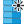#Generate Data (ARIMA) (Time Series)

## Synopsis

This operator generates a time series from an ARIMA process.

## Description

The process is defined by auto-regressive terms and moving-average terms, which defíne how strongly previous values of the time series influence the next values. The result of the operator is a single attribute that includes the time series.

## Differentiation

###Generate Data

This operator also generates a new ExampleSet. It offers many different generating functions and can generate ExampleSets with a label attribute.

## Output

•arima (Data Table)

ExampleSet which has only one attribute that represents the ARIMA time series.

## Parameters

• name_of_new_time_series_attribute

This parameter sets the name of the new time series attribute which is returned.

Range:
• coefficients_of_the_auto-regressive_terms

This parameter list specifies the coefficients of the auto-regressive terms.

Range:
• coefficients_of_the_moving-average_terms

This parameter list specifies the coefficients of the moving-average terms.

Range:
• constant

This parameters sets a starting point for the ARIMA process.

Range:
• standard_deviation_of_the_innovations

This parameter sets the standard deviation of the innovations. It controls the amount of variation that is added to each new data point.

Range:
• length

This parameter is the final length of the generated time series. It is the number of examples of the new ExampleSet.

Range:
• use_local_random_seed

This parameter indicates if a local random seed should be used. If selected a local seed is used specifically for this operator.

Range:
• local_random_seed

If the use local random seed parameter is checked this parameter determines the local random seed.

Range:

## Tutorial Processes

### Generating a sample ARIMA process

Simple process that generates an ARIMA time series.